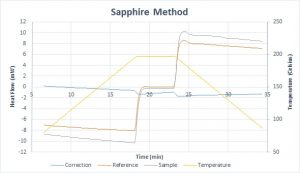The heat capacity of a material is a crucial property to consider when determining its functionality in certain applications. The typical SI unit of heat capacity (Cp) is Joules per gram Kelvin ($\frac{J}{gK}$). It is a measurement of how sensitive a material is to changes in temperature due to the flow of heat energy into the material. A material with a higher heat capacity requires more joules of energy to heat one gram by one degree Kelvin.

A material’s heat capacity is most commonly calculated using a differential scanning calorimeter (DSC). DSCs measure heat flow (mW) into a material, which is the energy transferred (mJ) per second. It plots the difference in heat energy between an empty reference and a sample kept at the same temperature. The MCL has two DSC-capable machines: the NETZSCH 3500 Sirius, and the TA Instruments SDT 650.

It is possible to calculate a sample’s unknown heat capacity by analyzing the difference in heat flow between a sample with a well-known heat capacity and the sample. A method available in the MCL is the sapphire method. Sapphire’s well-researched heat capacity across a large range of temperatures qualifies it as a suitable reference material, hence the method’s name.

The sapphire method is done in four steps. As a preliminary basis for accuracy in the sapphire method, DSC scans should be performed in immediate succession of one another. The scans should maintain identical testing conditions to one another. The reference and sample crucibles should be reused for each of the three scans. The designed temperature program should reach temperatures beyond the range of significance for the unknown test material, and should be identical for each scan.

### Correction Step

The first step is to perform a baseline or correction scan. A DSC correction scan is performed with both empty sample and reference crucibles to evaluate possible fluctuations in the DSC scans. These fluctuations can vary on a daily basis due to changes in barometric pressure, humidity, and surrounding laboratory operations.

It is worthwhile to note that the MCL’s SDT 650 is a dual-capability machine – TGA and DSC. Its geometry, which is necessary to measure both heat flow and mass change signals, introduces error to this step of the analysis. A buoyancy effect occurs within the furnace chamber as the surrounding purge gasses are heated and move around (convection). With empty, unweighted sample and reference crucibles, the moving gas will move the arms holding the crucibles. This movement causes undue noise, making it  harder to determine a correct heat capacity at higher temperatures.

### Sapphire Calibration

The second step is a calibration measurement with the sapphire, which is performed by referencing the previous correction scan. This is done with a baseline-corrected measurement, such as the correction+sample option on our NETZSCH DSC. The crucible parameters and temperature programs should be identical between the Correction Step (above) and the Sapphire Calibration. In this test, a pan holding a sapphire sample will be measured with an empty reference.

### Unknown Sample

The third step is a sample measurement with the sample of unknown Cp. Like step 2, this is a baseline-corrected scan, and should be run with the first step subtracted as it goes. The sample crucible will contain the unknown material and will be run against an empty crucible.

### Data Analysis

The final step is data evaluation to determine the heat capacity of the unknown material. The unknown heat capacity can be determined with the following equation:$Cp_s = \frac{H}{h}*\frac{m_r}{m_s}*Cp_r$

• Cps: unknown heat capacity of the test sample
• H: difference between sample and zero heat flow (baseline scan)
• h: difference between reference and zero heat flow (baseline scan)
• mr: mass of sapphire reference
• ms: mass of test sample
• Cpr: heat capacity of the sapphire reference

As an example, the sapphire method was performed on two sapphire samples, treating one as the unknown. You can see the three different tests: the empty correction scan in blue near the zero-heat flow line, the sapphire reference in orange, and the unknown test sample in gray.